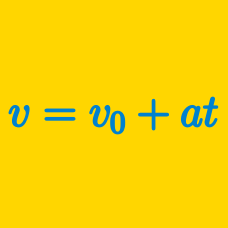Classical Mechanics

# Position, Velocity, and AccelerationSuppose that the displacement $$s$$ of an aeroplane can be expressed as a function of time $$t$$ in the following way: $s(t) = 8 t^2 + 10 t .$ What is the magnitude of the acceleration of the object?

Point $$P$$ moves along the $$x$$-axis in such a way that its position is $$x(t)=6-2t,$$ where $$t$$ denotes elapsed time. If $$t$$ has changed from $$0$$ to $$12,$$ what is the average velocity of point $$P?$$

At 5:30 pm, Stacey was driving her car at a speed of $$30\text{ mph}$$ on a straight avenue. Suddenly she notices that she had forgotten to bring her purse, so she immediately makes a U-turn and heads back to her house. Now she looks at her watch, which says 6:00 pm. If her current speed is $$40\text{ mph},$$ what is the magnitude of her average acceleration from 5:30 to 6:00 pm?

John is jogging from his home to the park, and back. If the distance between his home and the park is $$700$$ yards and John has jogged for a total of $$14$$ minutes, what is his average velocity, $$\vec{v}$$?

I'm driving along at a constant speed in my 3-meter-long car when I notice that I am accidentally driving through a red light. If the rear end of my car passes under the light 0.3 seconds after the front end of my car, how fast am I driving in m/s?

×

Problem Loading...

Note Loading...

Set Loading...